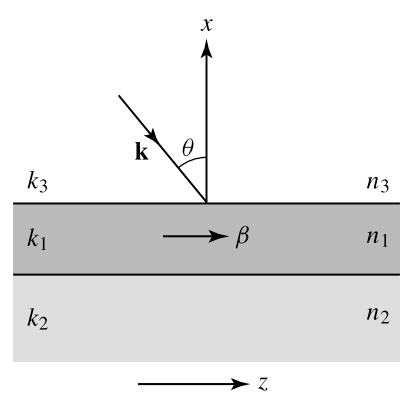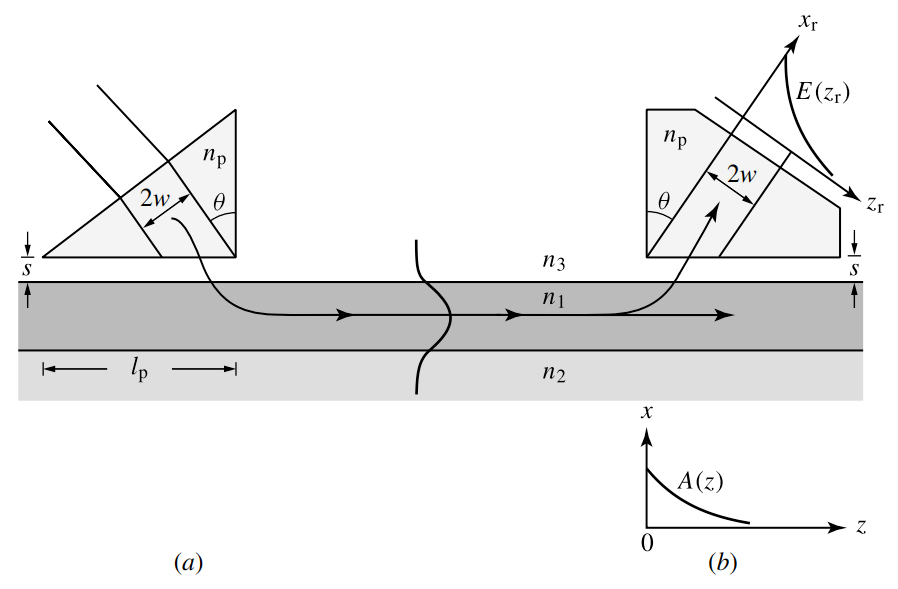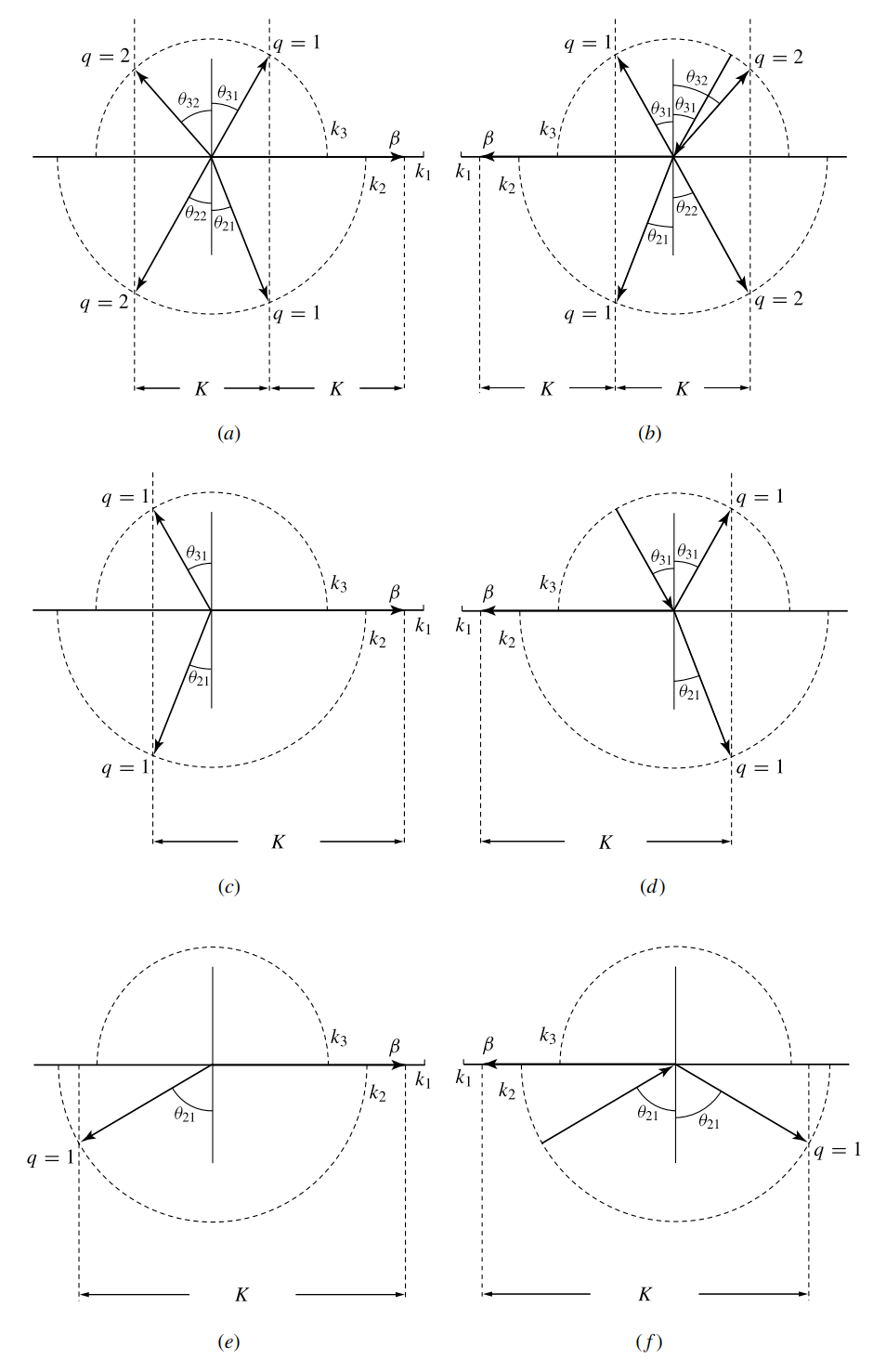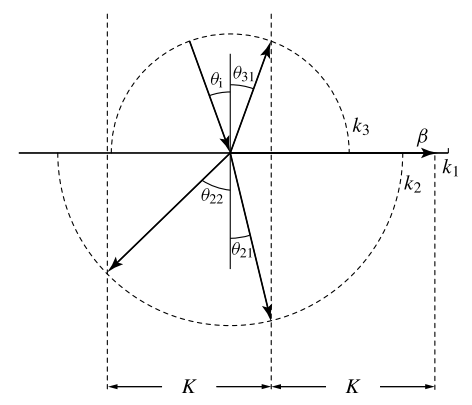# Surface Input and Output Couplers

This is a continuation from the previous tutorial - Directional Couplers.

In a system, it is always necessary to couple light from sources, such as lasers or light emitting diodes, to transmission components, which are usually dielectric waveguides or fibers, to various functional devices, such as optical switches, power dividers, amplifiers, and modulators, possible through transmission components again, and ultimately to photodetectors.

The approaches to coupling light in and out of optical waveguides, including fibers, are basically classified into two categories: (1) surface coupling, also called longitudinal coupling, and (2) end coupling, also called end-fire coupling or transverse coupling.

The first approach relies on the coupling of an optical wave in or out of a waveguide through the longitudinal surface of the waveguide. In the second approach, the optical wave is coupled directly through an exposed cross section at one end of the waveguide. In this tutorial, we examine the surface couplers.

From the viewpoint of coupled-mode theory, coupling of an optical wave through the longitudinal surface of a waveguide into a guided mode is an effect of coupling between the radiation modes of the waveguide and the guided mode. Any unguided propagating field such as that of the incident optical wave to be coupled into the waveguide can be expanded in terms of the radiation modes of the waveguide in the form of (23) and (24) [refer to the coupled-mode theory tutorial] with the summation replaced by integration over all radiation modes.

For efficient coupling, the phase-matching condition has to be satisfied, as we have seen time and again in the discussions of the preceding tutorials. Phase matching is not possible, however, without some special arrangements to perturb the system because the longitudinal propagation constant of any radiation mode is always smaller than that of the guide mode, as discussed in the waveguide modes tutorial and illustrated in figure 3 [refer to the waveguide modes tutorial]. This difficulty can be seen from the illustration in figure 10 below.Figure 10. Illustration of the phase mismatch between a guided mode and a free-propagating field incident at an angle $$\theta$$ on the surface of the waveguide.

For a beam incident on the surface of the guiding layer at an angle $$\theta$$, the largest propagation constant is that of a plane wave, $$k=k_3$$. Its longitudinal propagation constant is the $$z$$ component, which satisfies

$\begin{equation}\tag{63}k_z=k_3\sin\theta\lt k_3\lt\beta\end{equation}$

where $$\beta$$ is the propagation constant of a guide mode. By the same argument and by the reciprocity theorem for electromagnetic waves, it is equally impossible to couple the field in a guided mode out of the waveguide through the surface of the waveguide without some special arrangements.

The task of a surface coupler is to change this situation and to accomplish phase matching so that a radiation field can be coupled to the guide mode. Naturally, the same coupler can be used as an output coupler to couple a guided field out to a radiation field.

Prism Couplers

One approach to accomplishing phase matching is to use a prism of high index of refraction, $$n_p$$, as a surface coupler, as shown in figure 11 below.Figure 11. (a) Input and (b) output coupling using prism couplers.

For this arrangement, the cover of the waveguide is usually air or some low-index fluid filling the gap $$s$$ between the prism and the waveguide core. For $$k_p=n_p\omega/c$$, the phase-matching condition is then

$\begin{equation}\tag{64}k_p\sin\theta=\beta\end{equation}$

which can be accomplished if

$\begin{equation}\tag{65}n_p\gt n_1\gt n_3\qquad\text{and}\qquad\theta\gt\theta_c=\sin^{-1}\frac{n_3}{n_p}\end{equation}$

Note that the angle $$\theta$$ is now measured inside the prism. It is necessary that the total internal reflection occurs for the incident field inside the prism. As a result, the field does not propagate freely in the gap between the prism and the waveguide core. Then, the difficulty of phase matching due to (63) can be avoided. The total internal reflection results in an exponentially decaying evanescent field in the gap between the prism and the waveguide. Coupling to the waveguide mode occurs through optical tunneling when this evanescent field overlaps with the field of a guided mode if the phase-matching condition in (64) is satisfied.

Example 6

A rutile prism is used for surface coupling of optical waves at $$\lambda=1$$ μm into the guided modes of the waveguide described in the first example in the step-index planar waveguides tutorial. Rutile is a positive uniaxial crystal that has $$n_o$$ = 2.4585 and $$n_e$$ = 2.7495 at $$\lambda=1$$ μm. The optical wave to be coupled into the waveguide is incident on the prism surface at an incident angle of $$\theta_i$$, as shown in figure 12 below. The prism is cut with an angle of $$\zeta=45^{\circ}$$ and with its optical axis perpendicular to the plane of incidence as shown. What are the polarization of the incident optical wave and the angle $$\theta_i$$ for coupling into the $$\text{TE}_0$$ mode? What are they for coupling into the $$\text{TM}_0$$ mode?

The propagation constants for the $$\text{TE}_0$$ and $$\text{TM}_0$$ modes are $$\beta_\text{TE}=10.8432$$ μm-1 and $$\beta_\text{TM}=10.7800$$ μm-1, both found in the first example in the step-index planar waveguides tutorial. At $$\lambda=1$$ μm in rutile, $$k_p^o=2\pi n_0/\lambda=15.6187$$ μm-1 for the ordinary wave and $$k_p^e=n_e/\lambda=17.2756$$ μm-1 for the extraordinary wave. The optical wave is incident to the prism from the air with $$n_3=1$$.

For coupling into the $$TE_0$$ mode, the incident wave has to be TE polarized, in a direction perpendicular to the plane of incidence, in order to match the polarization of the guide $$TE_0$$ mode. This polarization is the extraordinary polarization in the given rutile prism. Therefore, $$k_p^e$$ is used for $$k_p^e\sin\theta=\beta_{TE}$$ to find that $$\theta=38.87^{\circ}$$ with the given parameters. We check that the critical angle $$\theta_c^e=\sin^{-1}(n_3/n_e)=21.33^{\circ}\lt\theta$$, as expected. From figure 12, we find that the angle of refraction $$\theta_t$$ for the wave transmitted into the prism is $$\theta_t=\zeta-\theta=6.13^{\circ}$$. Therefore, the angle of incidence is

$\begin{equation}\theta_i=\sin^{-1}\left(\frac{n_e}{n_3}\sin\theta_t\right)=17.07^{\circ}\end{equation}$

For coupling into the $$TM_0$$ mode, the incident wave has to be TM polarized, in the plane of incidence, in order to match the polarization of the guided $$TM_0$$ mode. This polarization is the ordinary polarization in the given rutile prism. Therefore, $$k_p^o$$ is used for $$k_p^o\sin\theta=\beta_{TM}$$ to find that $$\theta=43.65^{\circ}$$ with the given parameters. We check that the critical angle $$\theta_c^o=\sin^{-1}(n_3/n_o)=23.72^{\circ}\lt\theta$$, as expected. We then find that $$\theta_t=\zeta-\theta=1.35^{\circ}$$. Therefore, the angle of incidence is

$\begin{equation}\theta_i=\sin^{-1}\left(\frac{n_o}{n_3}\sin\theta_t\right)=3.36^{\circ}\end{equation}$

The coupling efficiency and other characteristics of the prism coupler can be analyzed with coupled-mode theory or other approaches of field analysis such as leaky-wave analysis. The mathematics is quite involved; therefore, we only discuss some key characteristics without detailed derivations.

It is more straightforward to understand the output coupling from a guided mode to the radiation field through a prism coupler shown in figure 11(b) above rather than the input coupling shown in figure 11(a) above. We thus consider the output coupler first.

For this purpose, we take $$z=0$$ to be at the edge of the output-coupling prism. For a guided mode that has an amplitude of $$A(0)$$ at $$z=0$$, the analysis using coupled-mode theory or leaky-wave theory indicates that the amplitude distribution along the $$z$$ direction for $$z\gt0$$ is

$\begin{equation}\tag{66}A(z)=A(0)\text{e}^{-\alpha_rz}\end{equation}$

where $$\alpha_r$$ is the radiation decay constant. The field coupled out to the prism has a pattern directly proportional to $$A(z)$$. For convenience, we assume that the prism is cut in such a manner that the output radiation field is normal to the exit surface of the prism as shown in figure 11(b) above. Then the output field has the following transverse pattern:

$\tag{67}E_\text{out}(z_r)=\begin{cases}0,\qquad&\text{for}\qquad\;z_r\lt0\\E_0\exp(-\alpha_rz_r/\cos\theta),&\text{for}\qquad\;z_r\gt0\\\end{cases}$

where $$z_r=z\cos\theta$$ is the coordinate transverse to the direction of propagation of the output field. The radiation decay constant has a complicated form but has the following key characteristics:

$\tag{68}\alpha_r\propto\frac{1}{d_\text{eff}}\text{e}^{-2\gamma_3s}$

where $$d_\text{eff}$$ is the effective waveguide thickness, $$d_\text{eff}=d_\text{E}$$ for a TE mode and $$d_\text{eff}=d_\text{M}$$ for a TM mode, and $$\gamma_3$$ is the transverse mode field parameter in the cover region defined by (52) in the step-index planar waveguides tutorial.

When the length of the prism facing the waveguide is $$l_p$$, the maximum output-coupling efficiency is

$\tag{69}\eta_\text{out}=1-\text{e}^{-2\alpha_rl_p}$

which can be obtained from (66) above. If $$l_p$$ is sufficiently long that $$\alpha_rl_p\gg1$$, an output-coupling efficiency that is nearly 100% can be achieved.

For input coupling, the situation is slightly more complicated than that of output coupling. Owing to the reciprocity theorem, input coupling is jus the reverse of output coupling. Therefore, an input efficiency of nearly 100% can be accomplished if an input beam propagating reversely with respect to the output beam shown in figure 11(b) above has exactly the same exponentially decaying profile as that given in (67). In practice, however, most of the input fields do not have such a profile. As a result, the coupling efficiency is reduced by the overlap factor $$\Gamma$$ of the two field patterns:

$\tag{70}\eta_\text{in}=\Gamma\eta_\text{out}$

where

$\tag{71}\Gamma=\frac{\left[\displaystyle\int\limits_{-\infty}^{\infty}E_\text{out}^*(z_r)E_\text{in}(z_r)\text{d}z_r\right]^2}{\displaystyle\int\limits_{-\infty}^{\infty}|E_\text{out}(z_r)|^2\text{d}z_r\displaystyle\int\limits_{-\infty}^{\infty}|E_\text{in}(z_r)|^2\text{d}z_r}$

and $$E_\text{in}(z_r)$$ is the transverse pattern of the input field.

The overlap factor $$\Gamma$$ depends on the profiles of $$E_\text{in}$$ and $$E_\text{out}$$. For $$E_\text{out}$$ having a pattern as given in (67), $$\Gamma$$ = 80.1% if $$E_\text{in}$$ has a Gaussian profile and $$\Gamma$$ = 81.4% if $$E_\text{in}$$ has a rectangular profile.

Therefore, the maximum input-coupling efficiency is 80.1% for a Gaussian input beam and is 81.4% for a rectangular input beam profile. However, in order to obtain the maximum input-coupling efficiency, alignment is very critical. In addition to sending the beam exactly at an angle required by phase matching, the parallel position of the beam has to be such that the front boundary of the beam exactly intercepts the prism corner, as shown in figure 11(a) above. Also, the length $$l_p$$ of the prism has to be such that $$l_p\gt2w/\cos\theta$$ to intercept the entire beam and that $$\alpha_rl_p\gg1$$.

For a given input or output coupler, the coupling efficiency depends on $$\alpha_r$$. It can be seen from (68) that $$\alpha_r$$ depends sensitively on the spacing $$s$$ between the prism and the waveguide.

For efficiency coupling, $$s$$ typically has to be kept less than half the optical wavelength in the medium filling the gap. This small gap is usually accomplished by applying a large pressure to clamp the prism onto the waveguide. The sensitivity of the coupling efficiency to the variation of this gap requires critical adjustment to control coupling. This is one of the disadvantages of prism couplers.

Furthermore, for good phase matching and good alignment, the input beam has to be well collimated. Another disadvantage is that the prism material must have a refractive index higher than that of the waveguide material. This requirement is particularly difficult to meet for a waveguide of high refractive index, such as one based on a semiconductor.

The advantages of prism couplers are that they are noninvasive and that no permanent structures have to be fabricated on the waveguides. Another advantage is that it is easy to excite different waveguide modes selectively by choosing appropriate incident angles for phase matching.

Prism couplers can be used for channel waveguides as well as planar waveguides. Therefore, prism couplers are most often used in the characterization of optical waveguides.

Grating Couplers

Another approach to obtaining phase matching for the coupling between the radiation field and a guided mode is the use of a grating. Figure 13 below shows input and output coupling of a planar waveguide using a grating surface coupler.Figure 13. (a) Input and (b) output coupling using grating couplers.

Basically, the function of the grating is to provide an extra phase factor $$qK$$ in a manner similar to that of the grating waveguide couplers discussed in the grating waveguide couplers tutorial so that modes of different propagation constants can be phase matched and efficiently coupled.

However, because the radiation fields are not restricted to a single propagation direction, the situation here is more complicated than that of the coupling between guided modes discussed in the grating waveguide couplers tutorial. The radiation fields can exist simultaneously in both the cover and the substrate regions, and the grating can scatter the light into different diffraction orders.

Because the grating is periodic only in the $$z$$ direction, the extra phase factor $$qK$$ it provides is also only in the $$z$$ direction. Therefore, the phase-matching condition for a guided mode with a propagation constant $$\beta$$ is

$\tag{72}k_{iq,z}+qK=\beta,\qquad\;i=2,3$

or

$\tag{73}k_i\sin\theta_{iq}+qK=\beta\qquad\;i=2,3$

where $$i=2$$ for the radiation fields in the substrate region of refractive index $$n_2$$, $$i=3$$ for those in the cover region of refractive index $$n_3$$, and $$\theta_{iq}$$ is the incident angle of $$\mathbf{k}_{iq}$$.

Note that because $$k_i\sin\theta_{iq}=k_{iq,z}$$, the sign of $$\theta_{iq}$$ is positive if $$k_{iq,z}=\mathbf{k}_{iq}\cdot\hat{z}\gt0$$ and is negative if $$k_{iq,z}=\mathbf{k}_{iq}\cdot\hat{z}\lt0$$. Because $$k_1\gt\beta\gt{k_2}\gt{k_3}$$, phase matching is possible only for $$q\ge1$$ in (73).

Similar to the treatment of the prism coupler, we consider output coupling first, which is also more straightforward than input coupling.

The field of a guided mode incident upon the grating region can be coupled to radiation fields in different diffraction orders that satisfy the phase-matching condition given by (73). The result can be output with multiple beams, two beams, or one beam, as shown in figure 14(a), (c), and (e) below, respectively.Figure 14. Phase-matched coupling between a guide mode and radiation fields: (a), (c), and (e) illustrate the conditions for output coupling into multiple beams, two beams, and one beam, respectively, which are determined by the value of $$K$$; (b), (d), and (f) show the conditions for input coupling corresponding to the reverse of (a), (c), and (e), respectively.

For a given waveguide and a given guided mode, the values of $$k_1,k_2,k_3,\text{and }\beta$$ are all fixed. Therefore, the number of phase-matched output beams is simply determined by the grating wavenumber $$K$$ or, equivalently, the grating period $$\Lambda=2\pi/K$$.

Because $$n_2\gt{n_3}$$, a single-beam output as shown in figure 14(e) can be obtained on the substrate side by making $$K$$ so large, or $$\Lambda$$ so small, that phase matching is not allowed for any diffraction order in the cover region. A single-beam output on the cover side can be obtained in the same manner by making $$n_3\gt{n_2}$$.

Example 7

A first-order grating is fabricated on the surface of a polymer core layer of the waveguide described in the first example in the step-index planar waveguides tutorial for surface coupling of an optical wave of 1 μm wavelength at an incident angle of 20° into the forward-propagating $$\text{TE}_0$$ mode of the waveguide. What is the period of the grating? How many diffracted beams are found on the cover and substrate sides? What are the directions of the diffracted beams?

We have $$\beta=10.8432\,\mu{m}^{-1}$$, $$k_2=9.1106\,\mu{m}^{-1}$$, and $$k_3=6.2832\,\mu{m}^{-1}$$ found in the example. The condition for first-order ($$q=1$$) input coupling at an incident angle $$\theta_i$$ is $$k_3\sin\theta_i+K=\beta$$. For $$\theta_i$$ = 20°, we find that $$K=\beta-k_3\sin\theta_i=8.6942\,\mu{m}^{-1}$$. Therefore, the grating period is

$\Lambda=\frac{2\pi}{K}=722.7\,\text{nm}$

The diffracted beams are generated by output coupling of the guide mode field due to scattering by the grating. Therefore, they can be found by finding the phase-matched output coupling from the guided mode. On the cover side, the condition is $$k_3\sin\theta_{3q}+qK=\beta$$, which leads to

$6.2832\sin\theta_{3q}=10.8432-8.6942q$

This condition has only one solution: $$\theta_{31}$$ = 20° for $$q=1$$. Therefore, there is only one diffracted beam on the cover side. At $$\theta_{31}$$ = 20°, this beam is in the specular reflection direction of the incident beam as shown in figure 15 below.Figure 15. Phase-matching diagram of surface input coupling through a first-order grating.

On the substrate side, the condition is $$k_2\sin\theta_{2q}+qK=\beta$$, which leads to

$9.1106\sin\theta_{2q}=10.8432-8.6942q$

This condition has two solutions: $$\theta_{21}$$ = 13.64° for $$q=1$$, and $$\theta_{22}$$ = - 45.9° for $$q=2$$. Therefore, there are two diffracted beams on the substrate side, one from first-order diffraction and the other from second-order diffraction of the guide mode field by the grating. The same results can be obtained by graphic solution, as shown in figure 15.

Similar to the output coupling with a prism coupler, the amplitude of the guide mode also decays exponentially in the grating region:

$\begin{equation}\tag{74}A(z)=A(0)\text{e}^{-\alpha_rz}\end{equation}$

where $$z=0$$ marks the beginning of the grating, as shown in figure 13(b) above.

In case there is more than one output beam, each output beam also has a pattern directly proportional to $$A(z)$$ along the surface of the grating in the $$z$$ direction. Because the direction of each output beam is determined by a different angle $$\theta_{iq}$$, the transverse field pattern across the cross section of an output beam is given by

$\tag{75}E_{iq}^{\text{out}}(z_{iq})=\begin{cases}0,\qquad&\text{for }z_{iq}\lt0\\E_{iq}(0)\exp(-\alpha_rz_{iq}/\cos\theta_{iq}),\qquad&\text{for }z_{iq}\gt0\end{cases}$

where $$z_{iq}$$ is the transverse coordinate along the cross section of the $$iq$$ output beam. Meanwhile, the total radiation decay constant is the sum of the radiation decay constants for the coupling of all existing output beams:

$\tag{76}\alpha_r=\sum_{i,q}\alpha_{iq}$

The radiation decay constants can be obtained from a coupled-mode or leaky-wave analysis. They also have a complicated form. However, their characteristics are very different from those of the prism coupler, as can be expected. The key characteristics are that for a grating of corrugation depth $$d_\text{g}$$,

$\tag{77}\alpha_{iq}\propto\frac{d_\text{g}^2}{d_\text{eff}},\qquad\text{if }d_\text{g}\le\frac{1}{\gamma_3}$

where $$d_\text{eff}$$ is the effective waveguide thickness used in (68), and that the values of $$\alpha_{iq}$$ saturate for $$d_\text{g}\gt\frac{1}{\gamma_3}$$. The dependence of $$\alpha_{iq}$$ on the indices $$i$$ and $$q$$ is determined by the shape and symmetry of the grating.

For a grating of length $$l_\text{g}$$, the output coupling efficiency to the beam of the indices $$i$$ and $$q$$ is

$\tag{78}\eta_{iq}^{\text{out}}=\frac{\alpha_{iq}}{\alpha_r}(1-\text{e}^{-2\alpha_rl_\text{g}})$

When $$\alpha_rl_\text{g}\gg1$$, all of the power in a guided mode is coupled out but is divided among the existing output beams propagating in different directioins.

Input coupling to a guided mode through a grating coupler can also be considered as the reverse of output coupling owing to the reciprocity theorem. However, again the situation is more complicated than that of the prism coupler because of the possibility of multiple diffraction orders. As a result, (1) there can be more than one incident direction for input coupling, and (2) the multiple-beam distribution for input coupling may look different from that of output coupling.

For example, in the case when phase matching is possible for multiple beams, as shown in figure 14(a), input coupling can be accomplished by sending in the bream in a reverse direction to any one of the output beams or by sending in more than one beam along any or all of these directions.

However, the pattern of the distribution of the diffracted beams, such as that shown in figure 14(b), looks different from that in the case of output coupling shown in figure 14 (a). Figures 14(d) and (f) show examples of input coupling corresponding to the output coupling shown in figure 14(c) and (e), respectively. In any event, if an input field is incident along the reverse path of an output beam of field $$E_{iq}$$ given by (75), the input coupling efficiency is simply

$\tag{79}\eta_{iq}^\text{in}=\Gamma_{iq}\eta_{iq}^\text{out}$

where

$\tag{80}\Gamma_{iq}=\frac{\left[\displaystyle\int\limits_{-\infty}^{\infty}E_{iq}^*(z_{iq})E_\text{in}(z_{iq})\text{d}z_{iq}\right]^2}{\displaystyle\int\limits_{-\infty}^{\infty}|E_{iq}(z_{iq})|^2\text{d}z_{iq}\displaystyle\int\limits_{-\infty}^{\infty}|E_\text{in}(z_{iq})|^2\text{d}z_{iq}}$

For an exponential beam profile of $$E_{iq}$$ given in (75), $$\Gamma_{iq}$$ = 80.1% if $$E_\text{in}$$ is Gaussian and $$\Gamma_{iq}$$ = 81.4% if it is rectangular.

In general, $$\alpha_{2q}\approx\alpha_{3q}$$ if the shape of the grating teeth is not highly asymmetric. Therefore, an output beam on the substrate side has about the same power as that of the same order on the cover side if both can be phase matched to the guided mode.

If this grating is used as an input coupler, then about one-half of the incident power will be lost before reduction by the overlap factor $$\Gamma_{iq}$$, resulting in a low coupling efficiency.

This shortcoming can be circumvented by using the one-beam coupler. One approach is that shown in figures 14(e) and (f). However, there are a few practical difficulties associated with this backward coupling through the substrate because the substrate tends to have a high refractive index close to that of the waveguide core. A more practical approach is to use a blazed grating with highly asymmetric teeth, such as those of saw-tooth shape, to cause the major portion of power to be coupled to a single order on the cover side. Alignment of the input beam is not extremely critical, but it is necessary to align the front boundary of the beam to intersect with the edge of the grating for maximum efficiency, as shown in figure 13(a) above.

In comparison to the prism coupler, grating couplers are compact and stable because they are integrated with the waveguide structure. They are compatible with the integration technology of photonic and optoelectronic devices. With proper design, they can be used as efficient input and output couplers in innovative applications, such as vertical output coupling from a semiconductor laser without conventional mirrors. Grating-coupled surface-emitting lasers based on this concept are discussed in later tutorials.

The next part continues with the Electro-Optic Effects tutorial